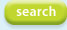# Bouncing Ball

## Description

Bouncing ball as a test for the Runge Kutta version with Variable time step. The ball bounces on a floor. During the bounce a much smaller step size is required. The RKV option takes care of this.

### Usage:

Run the model first with the standard set-up. Note that etot is (nearly) constant. By increasing the relative error, the accuracy can be controlled. Next, have a try with RK4 as the method, and note that the ball is launched by the floor.
```const G = 9.81;
t.min = 0;
t.max = 5.0;
t.step= 0.01;
t.sample = 0.01;
t.method = RKV;
t.relerror = 1.0E-5;
real    x(t), 	! position of ball
v(t), 	! velocity of ball
a(t), 	! acceleration of ball
f(t);	! force on ball
real	x0 = 1;
real    v0 = 0;
real  	c = 1000.0;	! stiffness of the floor
real  	m = 1.0;	! mass of the ball
real  	damp = 0.0;	! damping
real  	bounce = 0.0; 	! damping bouncing
real  	ekin(t), 	! kinetic energy
epot(t), 	! potential energy (gravity + spring)
etot(t); 	! total energy (should be constant)
module main;
begin
! forces on ball: gravity (-G*m) and spring stiffness of wall
f = -G*m + switch( x < 0.0 ? c*(-x) -bounce*v else 0.0) - damp*v;
a = f/m;
v = integ(a, v0);
x = integ(v, x0);
ekin = 0.5*m*v*v;
epot = m*G*x + switch(x < 0.0 ? 0.5*c*x*x else 0.0);
etot = ekin + epot;
end;
```